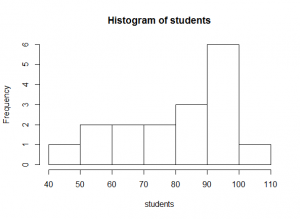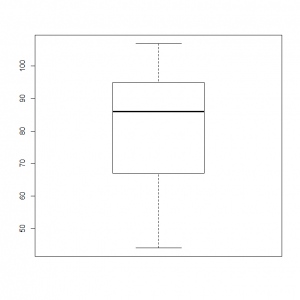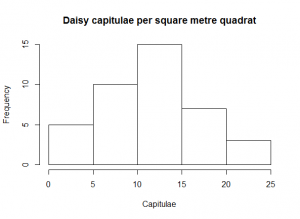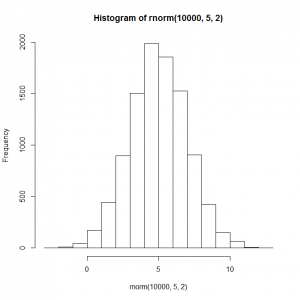# Descriptive statistics

Statistics can be used to describe a data set, or they can be used to infer how well a model fits a data set. In this post, we’ll look the the former kind of statistics, and how to extract them from R. The remaining posts in this series will mostly deal with statistical tests and modelling.

Descriptive statistics should usually give a measure of the central tendency of a data set (the mean and/or median), and of the variability of the data set (the range, quartiles, variance and/or standard deviation).

Consider the following data set:

# Number of students in lectures on successive days
students <-c(93, 95, 86, 76, 58, 77, 44, 107, 96, 95, 67, 67, 58, 87, 94, 100, 86)

Descriptive statistics should usually be accompanied by a graph that shows the data clearly to the reader in the most appropriate format for eye-balling. This set of data can be shown as a histogram in R using:

hist( students )The commonest ways of summarising a sample’s central tendency are to calculate the mean or the median of the sample.

A sample’s arithmetic mean (x̅) is the sum of all the values in the sample divided by the number, n, of items in the sample:$\bar{x} = \frac{\sum_{i=1}^{n} x_i }{ n }$
mean( students )
 81.52941

Although the arithmetic mean is frequently used to show the central tendency of a data set, you must take care in its interpretation. The mean number of students in lectures was 81, but you can see in the histogram above that on most days, the number of students fell between 90 and 100. This is because there is a large tail of low values in the distribution: the distribution is negatively skewed.

# Arithmetic mean, the hard way, using length() and sum()
total<-sum( students )
n<-length( students )
total/n
 81.52941

The usual measure of spread that goes with a mean, is the sample’s variance (s2). The variance is the sum of squares (SS) of the differences between each item in the sample and the mean, divided by the degrees of freedom remaining in the data set, i.e. you take each item in the data set, subtract the mean from it; square the result, add all of those squared differences up, and divide the total by the number of degrees of freedom.$s^2 = \frac{ \sum_{i=1}^{n} (x_i - \bar{x} )^2 }{ n-1 }$

Before you ask, the number of degrees of freedom in a data set is its size, n, minus the number of parameters (means, slopes, intercepts, etc.) you have estimated from it. If you estimate the mean of a set of data of size six, you remove one degree of freedom from it, and have only five left. The first five data points can take any value you like, but the value of the sixth point is constrained because the mean of all six points is known, and therefore the value of the sixth data point is also known.

var( students )
 306.7647

The standard deviation, s, is the square root of the variance. It has the advantage of having the same units as the mean, so is a useful measure of how far a ‘typical’ data point in your sample may lie from the mean of the sample.

sd( students )
 17.5147

Another measure of central tendency is often more useful when distributions are noticeably asymmetric: a sample’s median is the middle value in the ordered set of data (or the mean of the two middle samples if n is even).

median( students )
 86

The median is shown very clearly on a box-and-whisker-plot:

boxplot( students )In a box-and-whisker plot:

• The black bar represents the median.
• The box represents the interquartile range (25% to 75% of the data).
• The whiskers represent the full data range excluding any data more than 1.5 times larger than the upper quartile value, or 1.5 times smaller than the lower quartile.
• The dots represent any outlying data (i.e. those data excluded by the 1.5 criterion above). There are no outliers in this particular data set, but we’ll see them in later boxplots.

The range is the difference between the largest and smallest values, and the quantiles are the values at some fraction through the ordered data set, often at [0, ¼, ½ , ¾, 1] (quartiles) or at 1% intervals (percentiles).

range( students )
  44 107
quantile( students )
    0%  25% 50%  75% 100%
44   67  86   95  107
summary( students )
   Min. 1st Qu.  Median    Mean 3rd Qu.    Max.
44.00   67.00   86.00   81.53   95.00  107.00

# Exercises

Give summary statistics for the following data set

1. The file daisy_capitulae.csv contains data on the number of daisy capitulae (‘flowers’) in a larger number of quadrat samples in a field. Give some descriptive stats and a handy histogram to summarise it. Write this as prose: never just paste the raw text output of R into a document.
2. Create 10 000 normal data points using rnorm(), with mean 5 and standard deviation 2, and plot a histogram of them. help(rnorm) for the syntax.

1. In each square metre quadrat, the number of capitulae ranged from 2 to 24, with a median of 12.5. The mean number of capitulae was also 12.5(±5.6 s.d.). The histogram below show little evidence of skew, and the number of capitulae per square metre appears to be normally distributed.
daisy.capitulae<-read.csv( "H:/R/daisy_capitulae.csv" )
summary( daisy.capitulae )
Capitulae
Min.   : 2.00
1st Qu.: 9.00
Median :12.50
Mean   :12.47
3rd Qu.:15.25
Max.   :24.00
names(daisy.capitulae)
 "Capitulae"
sd( daisy.capitulae$Capitulae )  5.556182 hist( daisy.capitulae$Capitulae,
breaks = 5,
xlab   = "Capitulae",
main   = "Daisy capitulae per square metre quadrat"
)1. Ten thousand normal data points
hist( rnorm(10000, 5, 2) )Next up… Statistical testing.

This site uses Akismet to reduce spam. Learn how your comment data is processed.